How Cheenta works to ensure student success?
Explore the Back-Story

# Rectangle Problem | Geometry | PRMO-2017 | Question 13Try this beautiful Rectangle Problem from Geometry, from PRMO 2017.

## Rectangle Problem - Geometry - PRMO 2017, Question 13

In a rectangle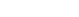is the midpoint of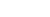is a point on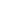such that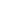is perpendicular to; and FE perpendicular to BD. Suppose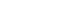. Find AB.

•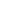•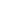•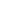### Key Concepts

Geometry

Triangle

Trigonometry

Answer:PRMO-2017, Problem 13

Pre College Mathematics

## Try with Hints

We have to find out the value of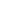. Join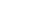.is perpendicular on.

Let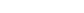since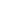is mid point of hypotenous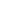of right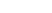, therefore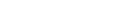Can you now finish the problem ..........

Therefore

Therefore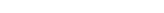and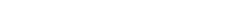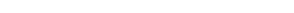But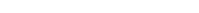Therefore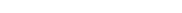Therefore in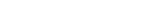Can you finish the problem........

Therefore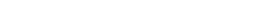## Subscribe to Cheenta at Youtube

Try this beautiful Rectangle Problem from Geometry, from PRMO 2017.

## Rectangle Problem - Geometry - PRMO 2017, Question 13

In a rectangleis the midpoint ofis a point onsuch thatis perpendicular to; and FE perpendicular to BD. Suppose. Find AB.

•••### Key Concepts

Geometry

Triangle

Trigonometry

Answer:PRMO-2017, Problem 13

Pre College Mathematics

## Try with Hints

We have to find out the value of. Join.is perpendicular on.

Letsinceis mid point of hypotenousof right, thereforeCan you now finish the problem ..........

Therefore

ThereforeandButThereforeTherefore inCan you finish the problem........

Therefore## Subscribe to Cheenta at Youtube

This site uses Akismet to reduce spam. Learn how your comment data is processed.

### Knowledge Partner